Download Presentation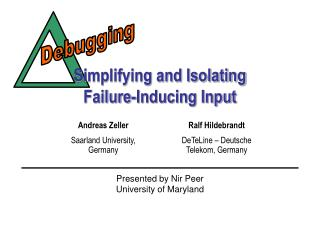Simplifying and Isolating Failure-Inducing Input

# Simplifying and Isolating Failure-Inducing Input - PowerPoint PPT Presentation

Andreas Zeller Saarland University, Germany. Ralf Hildebrandt DeTeLine – Deutsche Telekom, Germany. . Debugging. Simplifying and Isolating Failure-Inducing Input. Presented by Nir Peer University of Maryland. Introduction. Overview. Given some test case, a program fails.I am the owner, or an agent authorized to act on behalf of the owner, of the copyrighted work described.
Download Presentation## Simplifying and Isolating Failure-Inducing Input

An Image/Link below is provided (as is) to download presentation

Download Policy: Content on the Website is provided to you AS IS for your information and personal use and may not be sold / licensed / shared on other websites without getting consent from its author.While downloading, if for some reason you are not able to download a presentation, the publisher may have deleted the file from their server.

- - - - - - - - - - - - - - - - - - - - - - - - - - E N D - - - - - - - - - - - - - - - - - - - - - - - - - -
Presentation Transcript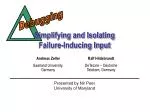Andreas Zeller

Saarland University, Germany

Ralf Hildebrandt

DeTeLine – Deutsche Telekom, Germany

Debugging

### Simplifying and IsolatingFailure-Inducing Input

Presented by Nir Peer

University of Maryland### Introduction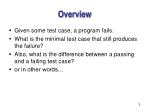Overview
• Given some test case, a program fails.
• What is the minimal test case that still produces the failure?
• Also, what is the difference between a passing and a failing test case?
• or in other words…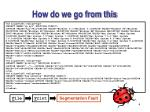How do we go from this

<td align=left valign=top><SELECT NAME="op sys" MULTIPLE SIZE=7><OPTION VALUE="All">All<OPTION VALUE="Windows 3.1">Windows 3.1<OPTION VALUE="Windows 95">Windows 95<OPTION VALUE="Windows 98">Windows 98<OPTION VALUE="Windows ME">Windows ME<OPTION VALUE="Windows 2000">Windows 2000<OPTION VALUE="Windows NT">Windows NT<OPTION VALUE="Mac System 7">Mac System 7<OPTION VALUE="Mac System 7.5">Mac System 7.5<OPTION VALUE="Mac System 7.6.1">Mac System 7.6.1<OPTION VALUE="Mac System 8.0">Mac System 8.0<OPTION VALUE="Mac System 8.5">Mac System 8.5<OPTION VALUE="Mac System 8.6">Mac System 8.6<OPTION VALUE="Mac System 9.x">Mac System 9.x<OPTION VALUE="MacOS X">MacOS X<OPTION VALUE="Linux">Linux<OPTION VALUE="BSDI">BSDI<OPTION VALUE="FreeBSD">FreeBSD<OPTION VALUE="NetBSD">NetBSD<OPTION VALUE="OpenBSD">OpenBSD<OPTION VALUE="AIX">AIX<OPTION VALUE="BeOS">BeOS<OPTION VALUE="HP-UX">HP-UX<OPTION VALUE="IRIX">IRIX<OPTION VALUE="Neutrino">Neutrino<OPTION VALUE="OpenVMS">OpenVMS<OPTION VALUE="OS/2">OS/2<OPTION VALUE="OSF/1">OSF/1<OPTION VALUE="Solaris">Solaris<OPTION VALUE="SunOS">SunOS<OPTION VALUE="other">other</SELECT></td><td align=left valign=top><SELECT NAME="priority" MULTIPLE SIZE=7>

<OPTION VALUE="--">--<OPTION VALUE="P1">P1<OPTION VALUE="P2">P2<OPTION VALUE="P3">P3<OPTION VALUE="P4">P4<OPTION VALUE="P5">P5</SELECT></td><td align=left valign=top><SELECT NAME="bug severity" MULTIPLE SIZE=7><OPTION VALUE="blocker">blocker<OPTION VALUE="critical">critical<OPTION VALUE="major">major<OPTION VALUE="normal">normal<OPTION VALUE="minor">minor<OPTION VALUE="trivial">trivial<OPTION VALUE="enhancement">enhancement</SELECT></tr></table>

File

Print

Segmentation Fault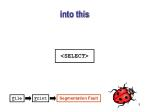into this

<SELECT>

File

Print

Segmentation Fault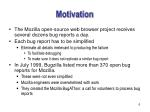Motivation
• The Mozilla open-source web browser project receives several dozens bug reports a day.
• Each bug report has to be simplified
• Eliminate all details irrelevant to producing the failure
• To facilitate debugging
• To make sure it does not replicate a similar bug report
• In July 1999, Bugzilla listed more than 370 open bug reports for Mozilla.
• These were not even simplified
• Mozilla engineers were overwhelmed with work
• They created the Mozilla BugAThon: a call for volunteers to process bug reports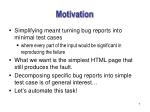Motivation
• Simplifying meant turning bug reports into minimal test cases
• where every part of the input would be significant in reproducing the failure
• What we want is the simplest HTML page that still produces the fault.
• Decomposing specific bug reports into simple test case is of general interest…
• Let’s automate this task!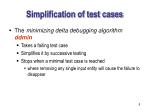Simplification of test cases
• The minimizing delta debugging algorithm ddmin
• Takes a failing test case
• Simplifies it by successive testing
• Stops when a minimal test case is reached
• where removing any single input entity will cause the failure to disappear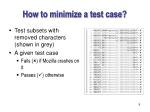How to minimize a test case?
• Test subsets with removed characters (shown in grey)
• A given test case
• Fails () if Mozilla crashes on it
• Passes () otherwise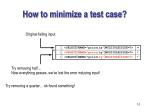How to minimize a test case?

Original failing input

Try removing half…Now everything passes, we’ve lost the error inducing input!

Try removing a quarter… ok found something!How to minimize a test case?

Try removing a quarter instead

OK, we’ve gotsomething!So keep it, and continue…

Good, carry on

Lost it!Try removing an eighth instead…How to minimize a test case?

Removing an eighth

Good, keep it!

Lost it!Try removing a sixteenth instead…

Great! we’re making progress

OK, now let’s see if removing single characters helps us reduce it even moreHow to minimize a test case?

Removing a single character

Reached a minimal test case!

Therefore, this should be ourtest case### FormalizationTesting for Change
• The execution of a program is determined by a a number of circumstances
• The program code
• Data from storage or input devices
• The program’s environment
• The specific hardware
• and so on
• We’re only interested in the changeable circumstances
• Those whose change may cause a different program behaviorThe change that Causes a Failure
• Denote the set of possible configurations of circumstances by R.
• Each rR determines a specific program run.
• This r could be
• a failing run, denoted by r
• a passing run, denoted by r
• Given a specific r
• We focus on the difference between r and some rR that works
• This difference is the change which causes the failure
• The smaller this change, the better it qualifies as a failure causeThe change that Causes a Failure
• Formally, the difference between r and r is expressed as a mapping  which changes the circumstances of a program run
• The exact definition of d is problem specific
• In the Mozilla example, applying d means to expand a trivial (empty) HTML input to the full failure-inducing HTML page.

Definition 1 (Change).A change is a mapping  : RR.The set of changes is C  R  R.The relevant change between two runs r,rR isa change C s.t. (r)  r.Decomposing Changes
• We assume that the relevant change d can be decomposed into a number of elementary changes d1,..., dn.
• In general, this can be an atomic decomposition
• Changes that can no further be decomposed

Definition 2 (Composition of changes).The change composition : CCC is defined as

(i j)(r) = i(j(r))Test Cases and Tests
• According to the POSIX 1003.3 standard for testing frameworks, we distinguish three test outcomes:
• The test succeeds (PASS, written here as )
• The test has produced the failure it was intended to capture (FAIL, written here as )
• The test produced indeterminate results (UNRESOLVED, written as ?)

Definition 3 (rtest).The function rtest: R{,,?} determines for a program run rRwhether some specific failure occurs () or not () or whether the test isunresolved (?).

Axiom 4 (Passing and failing run).rtest(r) =  and rtest(r) =  hold.Test Cases and Tests
• We identify each run by the set of changes being applied to r
• We define c as the empty set which identifies r (no changes applied)
• The set of all changes c = {1,2,...,n} identifiesr = (12...n)(r)

Definition 5 (Test case). A subset c c is called a test case.Test Cases and Tests

Definition 6 (test). The function test: 2 {,,?} is defined as follows:Let c c be a test case with c = {1,2,...,n}. Then

test(c) = rtest((12...n)(r))

holds.

Corollary 7 (Passing and failing test cases). The following holds:

test(c) = test() =  (“passing test case”)

test(c) = test({1,2,...,n}) =  (“failing test case”)### Minimizing Test CasesMinimal Test Cases
• If a test case c c is a minimum, no other smaller subset of c causes a failure
• But we don't want to have to test all 2|c| of c
• So we'll settle for a local minimum
• A test case is minimal if none of its subsets causes a failure

Definition 8 (Global minimum). A set c c is called the global minimum of c if:c'  c (|c'| < |c| test(c') )

holds.

Definition 9 (Local minimum). A test case c c is a local minimum of c or minimal if:c'  c (test(c') )

holds.Minimal Test Cases
• Thus, if a test case c is minimal
• It is not necessarily the smallest test case (there may be a different global minimum)
• But each element of c is relevant in producing the failure
• Nothing can be removed without making the failure disappear
• However, determining that c is minimal still requires 2|c| tests
• We can use an approximation instead
• It is possible that removing several changes at once might make a test case smaller
• But we'll only check if this is so when we remove up to n changesMinimal Test Cases
• We define n-minimality: removing any combination of up to n changes, causes the failure to disappear
• We're actually most interested in 1-minimal test cases
• When removing any single change causes the failure to disappear
• Removing two or more changes at once may result in an even smaller, still failing test case
• But every single change on its own is significant in reproducing the failure

Definition 10 (n-minimal test case). A test case c c is n-minimal if:c'  c (|c| - |c'| n  test(c') ) holds.

Consequently, c is 1-minimal if di  c (test(c - {di}) ) holds.The Delta Debugging Algorithm
• We partition a given test case c into subsets
• Suppose we have n subsets D1,...,Dn
• We test
• each Di and
• its complement i = c - DiThe Delta Debugging Algorithm
• Testing each Di and its complement, we have four possible outcomes
• Reduce to subset
• If testing any Di fails, it will be a smaller test case
• Continue reducing Di with n = 2 subsets
• Reduce to complement
• If testing any i = c - Di fails, it will be a smaller test case
• Continue reducing i with n - 1 subsets
• Why n - 1 subsets and not n = 2 subsets? (Maintain granularity!)
• Double the granularity
• DoneExample
• Consider the following minimal test case which consists of the changes 1, 7, and 8
• Any test case that includes only a subset of these changes results in an unresolved test outcome
• A test case that includes none of these changes passes the test
• We first partition the set of changes in two halves
• none of them passes the testExample
• We continue with granularity increased to four subsets
• When testing the complements, the set 2 fails, thus removing changes d3 and d4
• We continue with splitting 2 into three subsetsExample
• Steps 9 to 11 have already been carried out and need not be repeated (marked with *)
• When testing 2, changed 5 and 6 can be eliminated
• We reduce to 2 and continue with two subsetsExample
• We increase granularity to four subsets and test each
• Testing the complements shows the we can eliminate d2Example
• The next steps show that none of the remaining changes 1, 7, and 8 can be eliminated
• To minimize this test case, a total of 19 different tests was required### Case StudiesThe GNU C Compiler

#define SIZE 20

double mult(double z[], int n){ int i, j;

i = 0; for (j = 0; j < n; j++) { i = i + j + 1; z[i] = z[i] * (z + 1.0); } return z[n];}

void copy(double to[], double from[], int count){ int n = (count + 7) / 8; switch (count % 8) do { case 0: *to++ = *from++;case 7: *to++ = *from++;case 6: *to++ = *from++;case 5: *to++ = *from++;case 4: *to++ = *from++;case 3: *to++ = *from++;case 2: *to++ = *from++;case 1: *to++ = *from++; } while (--n > 0); return mult(to, 2);}

int main(int argc, char *argv[]){ double x[SIZE], y[SIZE]; double *px = x;

while (px < x + SIZE) *px++ = (px – x) * (SIZE + 1.0); return copy(y, x, SIZE);}

• This program (bug.c) causes GCC 2.95.2 to crash when optimization is enabled
• We would like to minimize this program in order to file a bug report
• In the case of GCC, a passing program run is the empty input
• For the sake of simplicity, we model change as the insertion of a single character
• r is running GCC with an empty input
• r means running GCC with bug.c
• each change di inserts the ith character of bug.cThe GNU C Compiler
• The test procedure would
• create the appropriate subset of bug.c
• feed it to GCC
• return  iff GCC had crashed, and  otherwise

77

755

377

188The GNU C Compiler
• The minimized code is
• The test case is 1-minimal
• No single character can be removed without removing the failure
• Even every superfluous whitespace has been removed
• The function name has shrunk from mult to a single t
• This program actually has a semantic error (infinite loop), but GCC still isn't supposed to crash
• So where could the bug be?
• We already know it is related to optimization
• If we remove the –O option to turn off optimization, the failure disappears

t(double z[],int n){int i,j;for(;;){i=i+j+1;z[i]=z[i]*(z+0);}return z[n];}The GNU C Compiler
• The GCC documentation lists 31 options to control optimization on Linux
• It turns out that applying all of these options causes the failure to disappear
• Some option(s) prevent the failure

–ffloat-store –fno-default-inline –fno-defer-pop

–fno-inline –finline-functions –fkeep-inline-functions

–fkeep-static-consts –fno-function-cse –ffast-math

–fcse-skip-blocks –frerun-cse-after-loop –frerun-loop-opt

–fgcse –fexpensive-optimizations –fschedule-insns

–fschedule-insns2 –ffunction-sections –fdata-sections

–fcaller-saves –funroll-loops –funroll-all-loops

–fmove-all-movables –freduce-all-givs –fno-peephole

–fstrict-aliasingThe GNU C Compiler
• We can use test case minimization in order to find the preventing option(s)
• Each di stands for removing a GCC option
• Having all di applied means to run GCC with no option (failing)
• Having no di applied means to run GCC with all options (passing)
• After seven tests, the single option -ffast-math is found which prevents the failure
• Unfortunately, it is a bad candidate for a workaround because it may alter the semantics of the program
• Thus, we remove -ffast-math from the list of options and make another run
• Again after seven tests, it turn out that -fforce-addr also prevents the failure
• Further examination shows that no other option prevents the failureThe GNU C Compiler
• So, this is what we can send to the GCC maintainers
• The minimal test case
• “The failure only occurs with optimization”
• “-ffast-math and -fforce-addr prevent the failure”Minimizing Fuzz
• In a classical experiment by Miller et al. several UNIX utilities were fed with fuzz input – a large number of random characters
• The studies showed that in the worst case 40% of the basic programs crashed or went into infinite loops
• We would like to use the ddmin algorithm to minimize the fuzz input sequences
• We examine the following six UNIX utilities
• NROFF (format documents for display)
• TROFF (format documents for typesetter)
• FLEX (fast lexical analyzer generator)
• CRTPLOT (graphics filter for various plotters)
• UL (underlining filter)
• UNITS (convert quantities)Minimizing Fuzz
• The following table summarizes the characteristics of the different fuzz inputs used

S = segmentation fault, A = arithmetic exceptionMinimizing Fuzz
• Out of the 6 16 = 96 test runs, the utilities crashed 42 times (43%)
• We apply our algorithm to minimize the failure-inducing fuzz input
• Our test function returns  if the input made the program crash
• or  otherwise